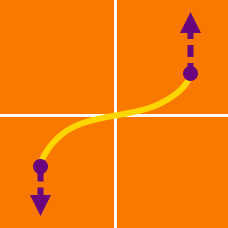Calculus

# Surface Area by Integration

The area of the surface obtained by revolving the curve $y = \sqrt{x}$, $4 \leq x \leq 7$, about the $x$-axis can be represented by $\frac{\pi}{6}(a \sqrt{a} - b \sqrt{b})$. What is the value of $a + b$?

The area of the plane $3x+2y+z = 30$, where $x \geq 0$, $y \geq 0$ and $z \geq 0$ can be represented by $a \sqrt{b}$, where $a$ and $b$ are positive integers and $b$ is not divisible by the square of a prime. What is the value of $a+b$?

What is the area of the surface obtained by revolving the curve $y=\sqrt{81-x^2}$ from $-2 \leq x \leq 2$ about the $x$-axis?

Let $S$ be the surface area of the solid obtained by rotating the curve $y = x^3$ $( 0 \leq x \leq 1)$ about the $x$-axis. If $S = \frac{\pi}{27}(a \sqrt{a} - 1)$, where $a$ is a positive integer, what is the value of $a$?

The area of the paraboloid $z = x^2 + y^2$, where $0 \leq z \leq 121$, can be represented by $\frac{\pi}{6} (a \sqrt{a} - 1)$. What is the value of $a$?

×